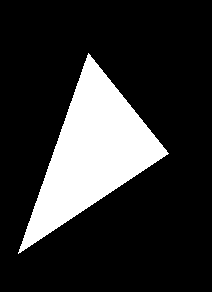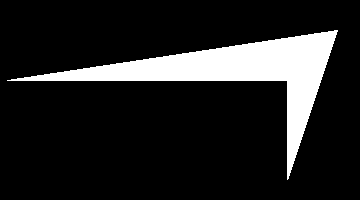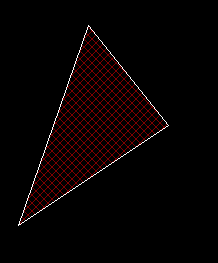# fillpoly() function in C

The header file graphics.h contains fillpoly() function which is used to draw and fill a polygon i.e. triangle, rectangle, pentagon, hexagon etc. It require same arguments as drawpoly().

Syntax :

```void fillpoly( int number, int *polypoints );

where,
number indicates (n + 1) number of points
where, n is the number of vertices in a
polygon. polypoints points to a sequence
of (n*2) integers.
```

Examples :

```Input : arr[] = {320, 150, 400, 250,
250, 350, 320, 150};
Output :Input : arr[] = {120, 250, 400, 250, 400,
350, 450, 200, 120, 250};
Output :```

Explanation : The declaration of fillpoly() contains two arguments: number indicates (n + 1) number of points where n is the number of vertices in a polygon.The second argument, i.e, polypoints points to a sequence of (n * 2) integers . Each pair of integers gives x and y coordinates of a point on the polygon. We specify (n + 1) points because first point coordinates should be equal to (n + 1)th to draw a complete figure.

Example 1: Drawing a triangle using fillpoly.
int arr[] = {320, 150, 400, 250, 250, 350, 320, 150};

Array arr contains coordinates of triangle which are (320, 150), (400, 250) and (250, 350). Note that last point(320, 150) in array is same as first.

Below is the implementation of fillpoly() function.

 `// C Implementation for fillpoly() ` `#include ` ` `  `// driver code ` `int` `main() ` `{ ` `    ``// gm is Graphics mode which is ` `    ``// a computer display mode that ` `    ``// generates image using pixels. ` `    ``// DETECT is a macro defined in ` `    ``// "graphics.h" header file ` `    ``int` `gd = DETECT, gm; ` `    `  `    ``// coordinates for polygon ` `    ``int` `arr[] = {320, 150, 400, 250,  ` `                ``250, 350, 320, 150}; ` ` `  `    ``// initgraph initializes the ` `    ``// graphics system by loading a ` `    ``// graphics driver from disk ` `    ``initgraph(&gd, &gm, ``""``); ` ` `  `    ``// fillpoly function ` `    ``fillpoly(4, arr); ` ` `  `    ``getch(); ` ` `  `    ``// closegraph function closes the ` `    ``// graphics mode and deallocates ` `    ``// all memory allocated by ` `    ``// graphics system . ` `    ``closegraph(); ` ` `  `    ``return` `0; ` `} `

Output :

``````

Note : fillpoly() fills using current fill pattern and color which can be changed using setfillstyle.

Below is the program which uses setfillstyle() for filling a polygon.

 `// C Implementation for fillpoly() ` `// using setfillstyle() ` `#include ` ` `  `// driver code ` `int` `main() ` `{ ` `    ``// gm is Graphics mode which is ` `    ``// a computer display mode that ` `    ``// generates image using pixels. ` `    ``// DETECT is a macro defined in ` `    ``// "graphics.h" header file ` `    ``int` `gd = DETECT, gm; ` ` `  `    ``// coordinates of polygon ` `    ``int` `arr[] = {320, 150, 400, 250,  ` `                 ``250, 350, 320, 150}; ` ` `  `    ``// initgraph initializes the ` `    ``// graphics system by loading a ` `    ``// graphics driver from disk ` `    ``initgraph(&gd, &gm, ``""``); ` ` `  `    ``// setfillstyle function sets the ` `    ``// current fill pattern and fill color. ` `    ``setfillstyle(XHATCH_FILL, RED); ` ` `  `    ``// fillpoly function ` `    ``fillpoly(4, arr); ` ` `  `    ``getch(); ` ` `  `    ``// closegraph function closes the ` `    ``// graphics mode and deallocates ` `    ``// all memory allocated by ` `    ``// graphics system . ` `    ``closegraph(); ` ` `  `    ``return` `0; ` `} `

Output :

``````

Attention reader! Don’t stop learning now. Get hold of all the important DSA concepts with the DSA Self Paced Course at a student-friendly price and become industry ready.

My Personal Notes arrow_drop_upIn love with a semicolon because sometimes i miss it so badly)

If you like GeeksforGeeks and would like to contribute, you can also write an article using contribute.geeksforgeeks.org or mail your article to contribute@geeksforgeeks.org. See your article appearing on the GeeksforGeeks main page and help other Geeks.

Please Improve this article if you find anything incorrect by clicking on the "Improve Article" button below.

Article Tags :
Practice Tags :

1

Please write to us at contribute@geeksforgeeks.org to report any issue with the above content.